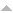## Publications

Export 82 results:
Sort by: Author Title [ Type] Year
Journal Article
Karlovich, Alexei Yu. "Singular integral operators with regulated coefficients in reflexive Orlicz spaces." Siberian Mathematical Journal. 38.2 (1997): 253-266.Website
Karlovich, Alexei Yu. "The Stechkin inequality for Fourier multipliers on variable Lebesgue spaces." Mathematical Inequalities and Applications. 18.4 (2015): 1473-1481. Abstract

We prove the Stechkin inequality for Fourier multipliers on variable Lebesgue spaces under some natural assumptions on variable exponents.

Karlovich, Alexei Yu, Yuri I. Karlovich, and Amarino B. Lebre. "Sufficient conditions for Fredholmness of singular integral operators with shifts and slowly oscillating data." Integral Equations and Operator Theory. 70.4 (2011): 451-483. AbstractWebsite

Suppose $$\alpha$$ is an orientation preserving diffeomorphism (shift) of $$\mathbb{R}_+=(0,\infty)$$ onto itself with the only fixed points $$0$$ and $$\infty$$. We establish sufficient conditions for the Fredholmness of the singular integral operator with shift $(aI-bW_\alpha)P_++(cI-dW_\alpha)P_-$ acting on $$L^p(\mathbb{R}_+)$$ with $$1 < p < \infty$$, where $$P_\pm=(I\pm S)/2$$, $$S$$ is the Cauchy singular integral operator, and $$W_\alpha f=f\circ\alpha$$ is the shift operator, under the assumptions that the coefficients $$a,b,c,d$$ and the derivative $$\alpha'$$ of the shift are bounded and continuous on $$\mathbb{R}_+$$ and may admit discontinuities of slowly oscillating type at $$0$$ and $$\infty$$.

Karlovich, Alexei Yu. "Toeplitz operators on abstract Hardy spaces built upon Banach function spaces." Journal of Function Spaces. 2017 (2017): Article ID 9768210, 8 pages.Website
Karlovich, Alexei Yu. "Wavelet bases in Banach function spaces." Bulletin of the Malaysian Mathematical Sciences Society (In Press).Website
Karlovich, Alexei Yu., and Eugene Shargorodsky. "When does the norm of a Fourier multiplier dominate its L-infinfty norm?" Proceedings of the London Mathematical Society. 118 (2019): 901-941.Website